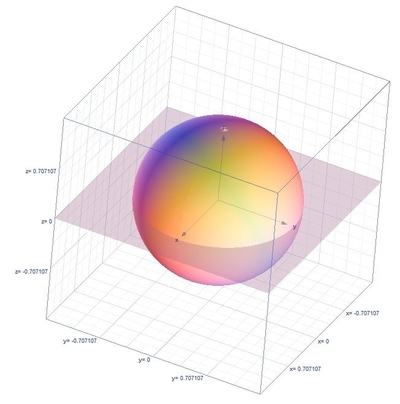# Evaluate Triple Integral: \int \int \int_E 16zdV; \ E=\{\text{Upper hemisphere of } x^2+y^2+z^2=1 \}

## Question:

Evaluate Triple Integral: {eq}\int \int \int_E 16zdV; \ E=\{\text{Upper hemisphere of } x^2+y^2+z^2=1 \}{/eq}

## Application of Triple Integrals:

Note that the triple integrals formula in solving the volume of the region is {eq}V=\int_{\alpha }^{\beta }\int_{h_{1}(\theta )}^{h_{2}(\theta )}\int_{u_{1}(r\cos \theta ,r\sin \theta )}^{u_{2}(r\cos \theta ,r\sin \theta )}r\:f\left ( r\cos \theta ,r\sin \theta ,z \right )dzdrd\theta {/eq} where the conversion formulas are the following {eq}r^{2}=x^{2}+y^{2},\:x=r\cos \theta ,\:y=r\sin \theta {/eq}.

## Answer and Explanation:

Below is the graph,First we need to find the limits of integrations, from the given equation when {eq}z=0 {/eq}

{eq}x^{2}+y^{2}=1 {/eq}

Thus, the limits are the following

{eq}0\leq \theta \leq 2\pi ,\:0\leq r\leq 1,\:0\leq z\leq \sqrt{1-r^{2}} {/eq}

Thus,

{eq}\displaystyle \int \int \int_E 16zdV = \int_{0}^{2\pi }\int_{0}^{1}\int_{0}^{\sqrt{1-r^{2}}}16zrdzdrd\theta {/eq}

Integrate with respect to {eq}z {/eq}

{eq}\displaystyle = \int_{0}^{2\pi }\int_{0}^{1}\left [ \frac{1}{2}z^{2} \right ]^{\sqrt{1-r^{2}}}_{0}16rdrd\theta {/eq}

{eq}\displaystyle = \int_{0}^{2\pi }\int_{0}^{1}\left ( \frac{-r^2+1}{2} \right )16rdrd\theta {/eq}

Integrate with respect to {eq}r {/eq}

{eq}\displaystyle = \int_{0}^{2\pi }\left [ 8\left(-\frac{r^4}{4}+\frac{r^2}{2}\right) \right ]^{1}_{0}d\theta {/eq}

{eq}\displaystyle = \int_{0}^{2\pi }2d\theta {/eq}

Integrate with respect to {eq}\theta {/eq}

{eq}\displaystyle = 2\left [ \theta \right ]^{2\pi }_{0} {/eq}

{eq}=4\pi {/eq}

#### Learn more about this topic:Volumes of Shapes: Definition & Examples

from GMAT Prep: Tutoring Solution

Chapter 11 / Lesson 9
45K